Altair® Panopticon

Steps:

1.     On the Visualization Settings pane, click the Spread variable. To associate other columns from the data table, drag and drop them to the Spread variable drop area. Select one to display the corresponding configuration pane.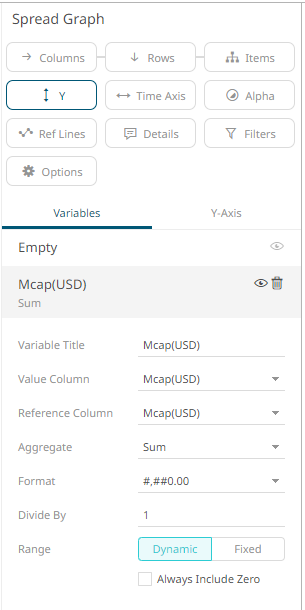2.     Enter the label of the Spread variable in the Variable Title field.

You can parameterize the variable title to support dynamic schema in the dashboards.

3.     You can also change the column to the be used as the Shape variable from the Value Column drop-down list.

4.     Select the Reference Column. The difference with the Value Column will be the basis if the variability or spread of the data is positive or negative.

For example:

 Value Column Reference Column Spread -7.2% -19.9% 12.7 (Positive) -8.1% -6.5% -1.6% (Negative)

5.     You can also specify an aggregation method in the Aggregate field.

The default is Sum.

The Spread variable also supports a number of other aggregate types:

·         If you set the aggregation method to Cumulative Sum or Cumulative Sum by Max, the Sort By drop-down list is enabled and displays a list of numeric data columns in the selected data table that can be used as the sorting column for the aggregate.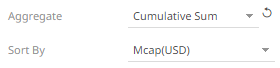Cumulative Sum By Max is, in practice, Cumulative Sum with a setting to use Max as the sort by aggregation.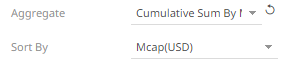·         If you set the aggregation method to Intercept or Slope, the Y Variable drop-down list is enabled and displays a list of numeric data columns in the selected data table that can be used as the Y-axis variable column for the aggregate.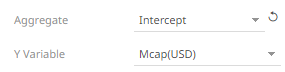·         If you set the aggregation method to Percent of Parent Reference, Percent of Total Reference, or Ratio of Sums, the Reference Column drop-down list is enabled and displays a list of numeric data columns in the selected data table that can be used as the reference column for the aggregate.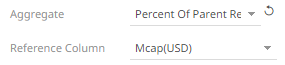·         If you set the aggregation method to Percent of Total Change, the Previous Values Column drop-down list is enabled and displays a list of numeric data columns in the selected data table that can be used as the previous column for the aggregate.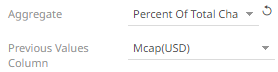·         If you set the aggregation method to Percentile, the Percentile field is displayed. Specify the value that can be used to calculate the value of the selected percentile.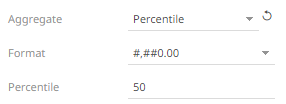·         If you set the aggregation method to Weighted Harmonic Mean, Weighted Mean, Weighted Population Variance, Weighted Stdev, Weighted Stdevp, Weighted Sum, or Weighted Variance, the Weight Column drop-down list is enabled and displays a list of numeric data columns in the selected data table that can be used as the weight column for the aggregate.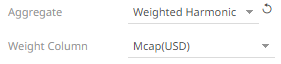6.     The Format field lets you specify the format that numbers will be displayed in. Panopticon uses the same formatting rules as Excel.

7.   Select the Divide By value to divide a number:

·         1

·         1000 (by a thousand)

·         10000

·         1000000 (by a million)

·         1000000000 (by a billion)

8.   The visible range for the Spread variable can either be calculated dynamically (the default, enabled Dynamic).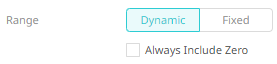Check the Always Include Zero box to let the axis scale start at zero, and grow to any number that may show up in the data.

Or set between predefined limits by clicking Fixed. This reveals the Min and Max text boxes and populates them with default values taken from the data set.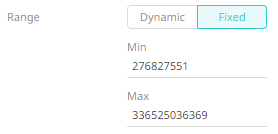9.   Click the Save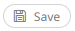icon on the toolbar.

When saved, the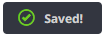notification is displayed.